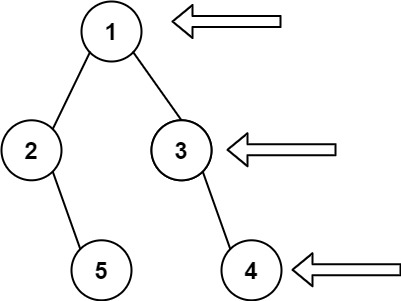# Binary Tree Right Side View

## 1: U-nderstand

Understand what the interviewer is asking for by using test cases and questions about the problem.

• Established a set (2-3) of test cases to verify their own solution later.
• Established a set (1-2) of edge cases to verify their solution handles complexities.
• Have fully understood the problem and have no clarifying questions.
• Have you verified any Time/Space Constraints for this problem?
• Can the input tree be Null?
• Yes, the root node can be Null.
• What is the space and time complexity?
• Time is `O(N)` and Space is `O(N)`.
``````HAPPY CASE
Input: root = [1,2,3,null,5,null,4]
Output: [1,3,4]````````````Input: root = [1,null,3]
Output: [1,3]

EDGE CASE
Input: root = []
Output: []``````

## 2: M-atch

Match what this problem looks like to known categories of problems, e.g. Linked List or Dynamic Programming, and strategies or patterns in those categories.

If you are dealing with Binary Trees some common techniques you can employ to help you solve the problem:

• Think about appropriate Tree Traversal: Pre-Order, In-Order, Post-Order, Level-Order
• Choosing a specific tree traversal helps identify the right node. Think level-order output.
• Store nodes within a HashMap to refer to later
• We don’t have a specific way of referring to previous nodes in a path that could be used in a HashMap. So, a HashMap would not help us as much in this context.
• Using Binary Search to find an element
• We are not working with a Binary Search Tree.
• Applying a level-order traversal with a queue
• Yes, this is the perfect match for this problem.

## 3: P-lan

Plan the solution with appropriate visualizations and pseudocode.

General Idea: Process the binary tree by level using a queue to repeatedly store and process all nodes from previous layer in queue.

``````1. Handle Null Tree
2. Create a results array to store rightmost node for each level.
3. Create a queue with root node and process until no more nodes in queue
a. Process the current number of nodes in queue to exclude new nodes added to queue.
b. Store the last node of each level and add children from each node to queue, to be processed in next level.
4. Return results``````

⚠️ Common Mistakes

• Choosing the wrong traversal type
• Try to walk through the problem by hand and see the order in which you are processing the nodes. This will clue you into the type of traversal necessary
• Will In-Order traversal tell you the rightmost node?
• Failing to recognize the need to store results during the processing of nodes. ## 4: I-mplement

Implement the code to solve the algorithm.

``````# Definition for a binary tree node.
# class TreeNode:
#     def __init__(self, val=0, left=None, right=None):
#         self.val = val
#         self.left = left
#         self.right = right
class Solution:
def rightSideView(self, root: Optional[TreeNode]) -> List[int]:
# Handle Null Tree
if not root:
return []

# Create a results array to store rightmost node for each level.
results = []

# Create a queue with root node and process until no more nodes in queue
queue = [root]
while queue:
# Process the current number of nodes in queue to exclude new nodes added to queue.
levelCount = len(queue)
for i in range(levelCount):
node = queue.pop(0)
# Store the last node of each level and add children from each node to queue, to be processed in next level.
if node.left:
queue.append(node.left)
if node.right:
queue.append(node.right)
if i == levelCount - 1:
results.append(node.val)

# Return results
return results``````
``````class Solution {
public List<Integer> rightSideView(TreeNode root) {
List<Integer> list = new ArrayList<Integer>();
// Handle Null Tree
if (root == null)
return list;

// Create a results array to store rightmost node for each level

// Create a queue with root node and process until no more nodes in queue
while (!queue.isEmpty()) {
// Process the current number of nodes in queue to exclude new nodes added to queue.
int size = queue.size();
TreeNode node = null;
while (size > 0) {
node = queue.poll();
if (node.left != null)
queue.offer(node.left);
if (node.right != null)
queue.offer(node.right);
size--;
}
// Store the last node of each level and add children from each node to queue, to be processed in next level.
}

// Return results
return list;
}
}``````

## 5: R-eview

Review the code by running specific example(s) and recording values (watchlist) of your code's variables along the way.

• Trace through your code with an input to check for the expected output
• Catch possible edge cases and off-by-one errors

## 6: E-valuate

Evaluate the performance of your algorithm and state any strong/weak or future potential work.

Assume `N` represents the number nodes in tree

• Time Complexity: `O(N)` because we need to visit each node in binary tree.
• Space Complexity: `O(N)` because we need to store each node's val into results.